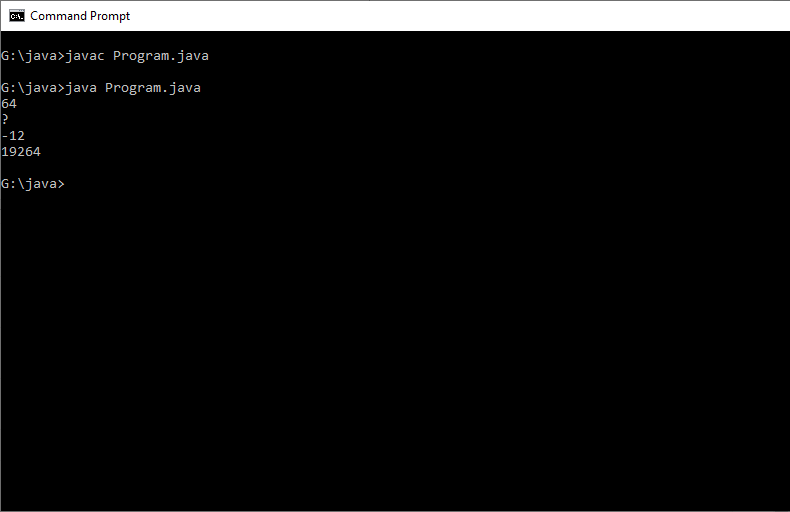# Explicit Type Conversion Example in Java

Write a program to perform explicit type conversion. Where implicit conversion is not possible.

#### Program or Solution

``` class Program{ public static void main(String args[]) { byte b = 10; char ch = 'C'; short num = 500; int i = 5000000; //during explicit conversion there will be loss in data  //for example 5000000 cannot accommodate in byte because the size is 1 byte (256 combinations only possible). // in this case 5000000 will be divided by 256 and remainder will be stored in byte (so 64) b=(byte)i; System.out.println(b); ch =(char)i; System.out.println(ch); b = (byte) num; System.out.println(b); num = (short)i; System.out.println(num); }} ```

#### Output#### Program Explanation

When you are assigning a larger type value to a variable of smaller type, then you need to perform explicit type casting.

During explicit conversion there will be loss in data. for example, 5000000 cannot accommodate in byte because the size is 1 byte (256 combinations only possible). In this case 5000000 will be divided by 256 and remainder will be stored in byte (so 64 us stored in b)